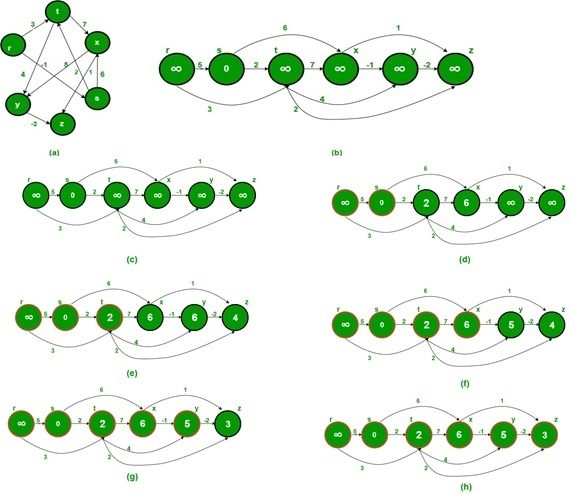# Shortest Path in a Directed Acyclic Graph

One weighted directed acyclic graph is given. Another source vertex is also provided. Now we have to find the shortest distance from the starting node to all other vertices, in the graph.

To detect Smaller distance, we can use another algorithm like Bellman-Ford for the graph with negative weight, for positive weight the Dijkstra’s algorithm is also helpful. Here for Directed Acyclic Graph, we will use the topological sorting technique to reduce complexity.## Input and Output

Input:
The cost matrix of the graph.
0   5  3 -∞ -∞ -∞
-∞  0  2  6 -∞ -∞
-∞ -∞  0  7  4  2
-∞ -∞ -∞  0 -1  1
-∞ -∞ -∞ -∞  0 -2
-∞ -∞ -∞ -∞ -∞  0

Output:
Shortest Distance from Source Vertex 1
Infinity 0 2 6 5 3

## Algorithm

topoSort(u, visited, stack)

Input: starting node u, the visited list to keep track, the stack.
Output: Sort the nodes in a topological way.

Begin
mark u as visited
for all vertex v, which is connected with u, do
if v is not visited, then
topoSort(v, visited, stack)
done
push u into the stack
End

shortestPath(start)

Input − The starting node.
Output − List of the shortest distance of all vertices from the starting node.

Begin
initially make all nodes as unvisited
for each node i, in the graph, do
if i is not visited, then
topoSort(i, visited, stack)
done

make distance of all vertices as ∞
dist[start] := 0
while stack is not empty, do
pop stack item and take into nextVert
if dist[nextVert] ≠∞, then
for each vertices v, which is adjacent with nextVert, do
if cost[nextVert, v] ≠∞, then
if dist[v] > dist[nectVert] + cost[nextVert, v], then
dist[v] := dist[nectVert] + cost[nextVert, v]
done
done

for all vertices i in the graph, do
if dist[i] = ∞, then
display Infinity
else
display dist[i]
done
End

## Example

#include<iostream>
#include<stack>
#define NODE 6
#define INF 9999

using namespace std;

int cost[NODE][NODE] = {
{0, 5, 3, INF, INF, INF},
{INF, 0, 2, 6, INF, INF},
{INF, INF, 0, 7, 4, 2},
{INF, INF, INF, 0, -1, 1},
{INF, INF, INF, INF, 0, -2},
{INF, INF, INF, INF, INF, 0}
};

void topoSort(int u, bool visited[], stack<int>&stk) {
visited[u] = true;       //set as the node v is visited
for(int v = 0; v<NODE; v++) {
if(cost[u][v]) {       //for allvertices v adjacent to u
if(!visited[v])
topoSort(v, visited, stk);
}
}

stk.push(u);       //push starting vertex into the stack
}

void shortestPath(int start) {
stack<int> stk;
int dist[NODE];

bool vis[NODE];
for(int i = 0; i<NODE;i++)
vis[i] = false;          // make all nodes as unvisited at first

for(int i = 0; i<NODE; i++)     //perform topological sort for vertices
if(!vis[i])
topoSort(i, vis, stk);

for(int i = 0; i<NODE; i++)
dist[i] = INF;       //initially all distances are infinity
dist[start] = 0;       //distance for start vertex is 0

while(!stk.empty()) {    //when stack contains element, process in topological order
int nextVert = stk.top(); stk.pop();

if(dist[nextVert] != INF) {
for(int v = 0; v<NODE; v++) {
if(cost[nextVert][v] && cost[nextVert][v] != INF){ if(dist[v] > dist[nextVert] +cost[nextVert][v])dist[v] = dist[nextVert] + cost[nextVert][v];
}
}
}
for(int i = 0; i<NODE; i++)
(dist[i] == INF)?cout << "Infinity ":cout << dist[i]<<" ";
}

main() {
int start = 1;
cout << "Shortest Distance From Source Vertex "<<start<<endl;
shortestPath(start);
}

## Output

Shortest Distance From Source Vertex 1
Infinity 0 2 6 5 3